# Algebraic equation facts for kids

Kids Encyclopedia Facts

In mathematics, an algebraic equation, also called polynomial equation over a given field is an equation of the form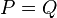$P = Q$

where P and Q are polynomials over that field, and have one (univariate) or more than one (multivariate) variables. For example: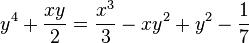$y^4+\frac{xy}{2}=\frac{x^3}{3}-xy^2+y^2-\frac{1}{7}$

is an algebraic equation over the rational numbers.

Two equations are called equivalent if they have the same set of solutions. This means that all solutions of the second equation must also be solutions of the first one and vice versa. The equation$P = Q$ is equivalent with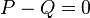$P-Q = 0$. So the study of algebraic equations is equivalent to the study of polynomials.

If an algebraic equation is over the rationals, it can always be converted to an equivalent one, where all the coefficients are integers. For example, in the equation given above, we multiply by 42 = 2·3·7 and group the terms in the first member. The equation is converted to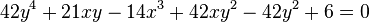$42y^4+21xy-14x^3+42xy^2-42y^2+6=0$

The solutions of an equation are the values of the variables for which the equation is true. But for algebraic equations there are also called roots. When solving an equation, we need to say in which set the solutions are allowed. For example, for an equation over the rationals, one can find solutions in the integers. Then, the equation is a diophantine equation. One may also look for solutions in the field of complex numbers. One can also look for solutions in real numbers.

Ancient mathematicians wanted the solutions of univariate equations (that is, equations with one variable) in the form of radical expressions, like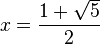$x=\frac{1+\sqrt{5}}{2}$ for the positive solution of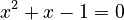$x^2+x-1=0$. The ancient Egyptians knew how to solve equations of degree 2 (that is equations in which the highest power of the variable is 2) in this manner. During the Renaissance, Gerolamo Cardano solved the equation of degree 3 and Lodovico Ferrari solved the equation of degree 4. Finally Niels Henrik Abel proved in 1824 that the equation of degree 5 and the equations of higher degree cannot always be solved by using radicals. Galois theory, named after Évariste Galois, were introduced to give criteria deciding if an equation is solvable using radicals.Algebraic equation Facts for Kids. Kiddle Encyclopedia.